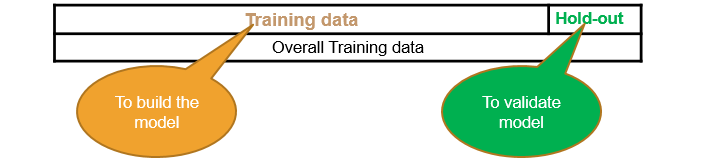• No products in the cart.

# 204.4.10 Cross Validation

##### Cross validating a model
`Link to the previous post : https://statinfer.com/204-4-9-model-bias-variance-tradeoff/`

## Cross Validation

We always build and train a model with a training dataset. With default parameters the fitting process optimizes the training data as well as possible. Introducing, a whole different sample to pre-built model won’t replicate the accuracy we expect form the model.

One way to solve this problem is cross validation. We can divide our dataset into training and testing samples. After fitting the model with training sample we can validate the accuracy on test sample. This method is called cross validation.

Commonly used Cross Validation techniques:

• Hold-Out data Cross Validation
• K-fold Cross Validation
• 10-fold Cross Validation
• Bootstrap Cross Validation

### Holdout Data Cross Validation

• The best solution is out of time validation. Or the testing error should be given high priority over the training error.
• A model that is performing good on training data and equally good on testing is preferred.
• We may not have the test data always. How do we estimate test error?
• We take the part of the data as training and keep aside some potion for validation. May be 80%-20% or 90%-10%
• Data splitting is a very basic intuitive method## LAB: Holdout Data Cross Validation

• Data: Fiberbits/Fiberbits.csv
• Take a random sample with 80% data as training sample
• Use rest 20% as holdout sample.
• Build a model on 80% of the data. Try to validate it on holdout sample.
• Try to increase or reduce the complexity and choose the best model that performs well on training data as well as holdout data.

Solution

In :
```#Splitting data into 80:20::train:test
X_train, X_test, y_train, y_test = train_test_split(X,y, train_size = 0.8)
```
In :
```#Defining tree parameters and training the tree
tree_CV = tree.DecisionTreeClassifier(criterion='gini',
splitter='best',
max_depth=20,
min_samples_split=2,
min_samples_leaf=1)
tree_CV.fit(X_train,y_train)
```
Out:
```DecisionTreeClassifier(class_weight=None, criterion='gini', max_depth=20,
max_features=None, max_leaf_nodes=None, min_samples_leaf=1,
min_samples_split=2, min_weight_fraction_leaf=0.0,
presort=False, random_state=None, splitter='best')```
In :
```#Training score
tree_CV.score(X_train,y_train)
```
Out:
`0.95631250000000001`
In :
```#Validation Accuracy on test data
tree_CV.score(X_test,y_test)
```
Out:
`0.85909999999999997`

Improving the above model:

In :
```tree_CV1 = tree.DecisionTreeClassifier(criterion='gini',
splitter='best',
max_depth=10,
min_samples_split=30,
min_samples_leaf=30,
max_leaf_nodes=30)
tree_CV1.fit(X_train,y_train)
```
Out:
```DecisionTreeClassifier(class_weight=None, criterion='gini', max_depth=10,
max_features=None, max_leaf_nodes=30, min_samples_leaf=30,
min_samples_split=30, min_weight_fraction_leaf=0.0,
presort=False, random_state=None, splitter='best')```
In :
```#Training score of this pruned tree model
tree_CV1.score(X_train,y_train)
```
Out:
`0.85914999999999997`
In :
```#Validation score of pruned tree model
tree_CV1.score(X_test,y_test)
```
Out:
`0.85624999999999996`

The model above is giving same accuracy on training and holdout data.

The next post is about k fold cross validation.

Link to the next post : https://statinfer.com/204-4-11-k-fold-cross-validation/

21st June 2017

### 0 responses on "204.4.10 Cross Validation"

#### Statinfer

Statinfer derived from Statistical inference is a company that focuses on the data science training and R&D.We offer training on Machine Learning, Deep Learning and Artificial Intelligence using tools like R, Python and TensorFlow